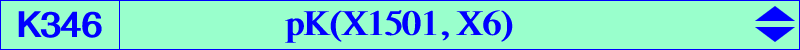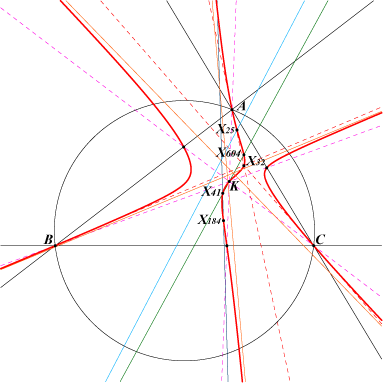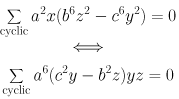X(6), X(25), X(31), X(32), X(41), X(184), X(604), X(2199), X(3172) harmonic associates of X(31)K346 is the isogonal transform of K184 i.e. the isogonal transform of the isotomic transform of the Thomson cubic K002. Hence, it is anharmonically equivalent to K002. See Table 21. More generally, K346 is the barycentric products X(1) x K175, X(3) x K445, X(4) x K576, X(6) x K002, X(25) x K099, X(31) x K034, X(32) x K184, X(184) x K647, hence all mentioned cubics are anharmonically equivalent. K346 is the locus of the poles of pKs meeting the circumcircle at the same points as the Thomson cubic K002. This is the case of K167 = pK(X184, X6) and K172 = pK(X32, X3). The locus of the pivots of such cubics is K002 itself and the locus of the isopivots is K172. In other words, for any P on the Thomson cubic, there is one point Ω on K346 such that pK(Ω, P) and K002 meet the circumcircle at the same six points. See Table 56. See a generalization in How pivotal cubics intersect the circumcircle.